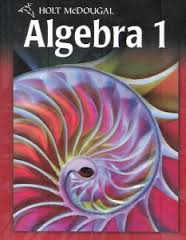Algebra 1 Quiz 5.2

12 Questions | Total Attempts: 665Settings• 1.
Which point is a solution of the inequality y > 2x + 3
• A.

(–2, –4)

• B.

(0, –1)

• C.

(5, 2)

• D.

(–3, 6)

• E.

None of these

• 2.
Which point is NOT a solution of the inequality y < –x + 3
• A.

(–2, –4)

• B.

(0, –1)

• C.

(4, –1)

• D.

(–5, 6)

• E.

All of these are solutions

• 3.
Which of the following describes the graph of y > –2x + 5?
• A.

The boundary line is dashed, and the shading is above the line.

• B.

The boundary line is dashed, and the shading is below the line.

• C.

The boundary line is solid, and the shading is above the line.

• D.

The boundary line is solid, and the shading is below the line.

• 4.
Which of the following describes the graph of y < –4?
• A.

The boundary line is dashed, and the shading is above.

• B.

The boundary line is dashed, and the shading is below.

• C.

The boundary line is dashed, and the shading is to the left.

• D.

The boundary line is dashed, and the shading is to the right.

• 5.
Write a system of linear inequalities to represent the graph below.
• A.

Not here

• 6.
Write a system of linear inequalities to represent the graph below.
• A.

Not here

• 7.
Which inequality is best represented by the graph below?
• A.

Not here

• 8.
Which inequality is best represented by the graph below?
• A.

Not here

• 9.
Which inequality is represented by the graph below?
• A.

Not here

Related TopicsBack to top